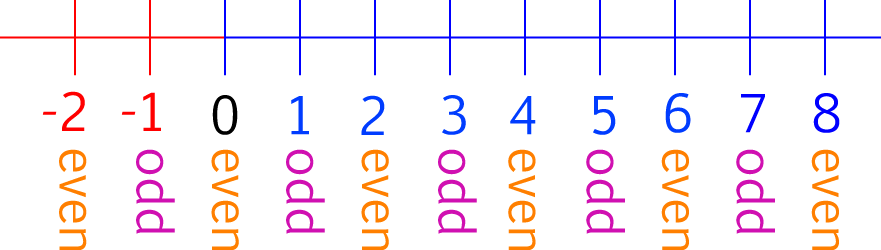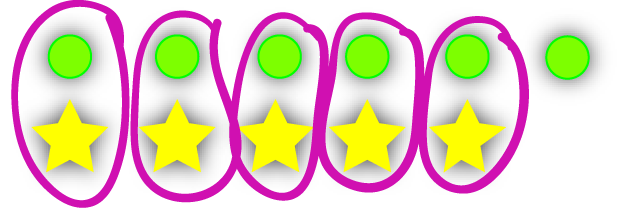# Splitting up the Whole NumbersAs you can see on the above number line, the whole numbers alternate between being odd and even. Every whole number is either odd or even.

## Even Numbers

Even numbers are whole numbers (integers) that can be divided evenly by $2$.There are 10 circles and stars in the above diagram. They split up into pairs with one circle and one star in each, a bit like people in a set at a bush dance. Each circle has a star as its dancing partner. $10$ is an even number because it can be divided up into pairs like this.

The even numbers include numbers like

$\dots,-8,-6,-4,-2,0,2,4,6,8,\dots$
They all have a final digit of
$0,2,4,6,$ or $8$

### Examples:

• The number $23,564$ is even. It ends in a $4$.
• $0$ is an even number
• $-256,276$ is also even. Its final digit is a $6$.
• $23,578,269,357$ is not even as its final digit is not $0,2,4,6$ or $8$.

## Odd Numbers

Odd numbers are the whole numbers that cannot be divided exactly by $2$. When you try to split an odd number of people up into dancing partners, there's always one left over.The picture above represents the number $11$. It shows a total of 6 circles and 5 stars. We can't divide it evenly up into circles and stars, so $11$ is an odd number. The poor circle on the right hand side is missing a dancing partner.

The odd numbers include:

$\dots,-7,-5,-3,-1,1,3,5,7,\dots$

Odd numbers end in one of the digits

$1,3,5,7,$ or $9$

### Examples:

• The number $23,593,235,789$ is odd. It ends in a $9$.
• $-273,595$ is an odd number. Its final digit is $5$
• $23,578,269,354$ is not odd as its final digit is not $1,3,5,7$ or $9$. In fact, it is even.

## Adding and Subtracting Odd and Even Numbers

What happens when we add and subtract odd and even numbers? We sometimes match up dancing partners, and sometimes don't. We might even take them away. There are rules telling us whether the result of an addition or subtraction is odd or even:

 Operation Result Example (even numbers are purplish-pink and odd numbers are orange). Even + Even Even 14 + 12 = 26 Odd + Odd Even 3 + 5 = 8 Odd + Even Odd 3 + 2 = 5 Even + Odd Odd 2 + 7 = 9

We get the same pattern when we replace the plus sign with a minus sign and subtract.

## Multiplying Odd and Even Numbers

What happens when we multiply odd and even numbers together? There's a list of rules that tell us whether the result is an odd or an even number:

 Operation Result Example (even numbers are purplish-pink and odd numbers are orange). Even $\times$ Even Even 4 $\times$ 2 = 8 Even $\times$ Odd Even 2 $\times$ 5 = 10 Odd $\times$ Even Even 3 $\times$ 2 = 6 Odd $\times$ Odd Odd 3 $\times$ 5 = 15

### Description

This mini book covers the core of Math for Foundation, Grade 1 and Grade 2 mathematics including

1. Numbers
3. Subtraction
4. Division
5. Algebra
6. Geometry
7. Data
8. Estimation
9. Probability/Chance
10. Measurement
11. Time
12. Money
13. and much more

This material is provided free of cost for Parent looking for some tricks for their Prekinder, Kinder, Prep, Year 1 and Year 2 children

### Learning Objectives

These lessons are for kids aged 4-8 with the core objective to expose their brains to concepts of addition, subtraction, division, algebra and much more.

Author: Subject Coach
You must be logged in as Student to ask a Question.